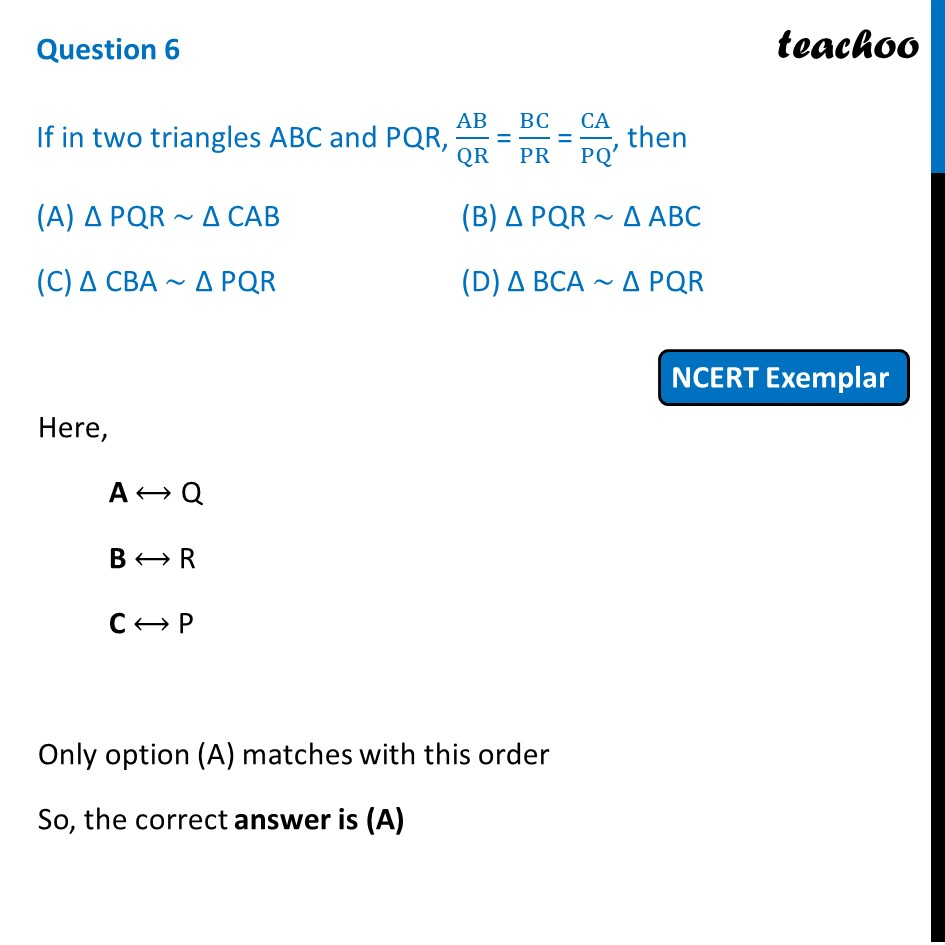## (C) ∆ CBA ~ ∆ PQR  (D) ∆ BCA ~ ∆ PQR1. Chapter 6 Class 10 Triangles (Term 1)
2. Serial order wise
3. NCERT Exemplar - MCQ

Transcript

Question 6 If in two triangles ABC and PQR, AB/QR = BC/PR = CA/PQ, then ∆ PQR ~ ∆ CAB (B) ∆ PQR ~ ∆ ABC (C) ∆ CBA ~ ∆ PQR (D) ∆ BCA ~ ∆ PQR Here, A ⟷ Q B ⟷ R C ⟷ P Only option (A) matches with this order So, the correct answer is (A)